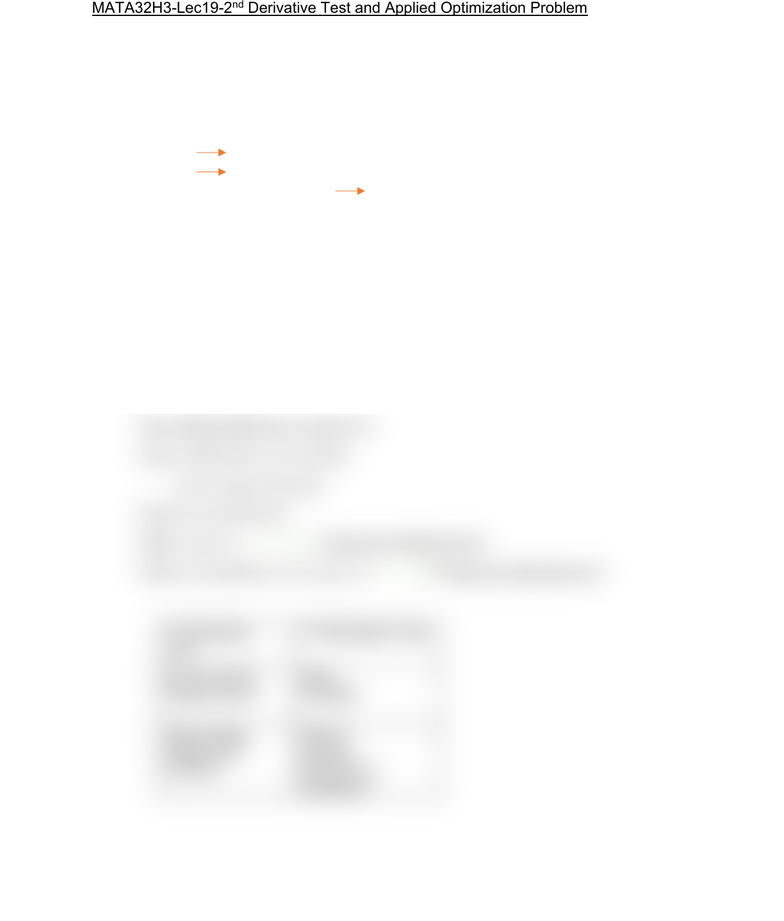Class Notes (1,100,000)
CA (630,000)
UTSC (30,000)
Mathematics (1,000)
MATA32H3 (200)
Lecture 19

# MATA32H3 Lecture Notes - Lecture 19: Maxima And MinimaPremium

Department
Mathematics
Course Code
MATA32H3
Professor
Karimian Pour, C.
Lecture
19

This preview shows half of the first page. to view the full 2 pages of the document.MATA32H3-Lec19-2nd Derivative Test and Applied Optimization Problem
2nd Derivative Test
Let y=f(x) be a function such that f’(c)=0
1. If f’(c)>0 local MIN at c
2. If f’(c)<0 local MAX at c
3. If f’(c)=0 or f’(c) is undefined 2nd derivative is of no help
Example:
(when the 2nd derivative is easier than the 1st)
Let y=f(x)=x^2e^-x
Find the critical point using 2nd Derivative Test for maximum/minimum
Solution:
f’(x)=2xe^-x +x^2e^-x (-1) = e^-x (2x-x^2)
= e^-x (2-x) x
The critical points are x=0 and x=2.
f’’(x)= -e^(2x-x^2) + e^-x (2-2x)
= e^-x (-2x+x^2+2-2x)
f’’(x)= e^-x (x^2-4x+2)
f’’(0)= 1 (2) = 2 f has local minimum at 0
f’’(2)= e^-2 (4-8+2) = e^-2 (-2) < 0 f has local maximum at 2
1st Derivative
Test
2nd Derivative Test
Do not need f’’
Always works
Easy…
Evaluate
Sign change
needs to be
checked
Need f’’
Can fail
NA if f’(c) is
undefined